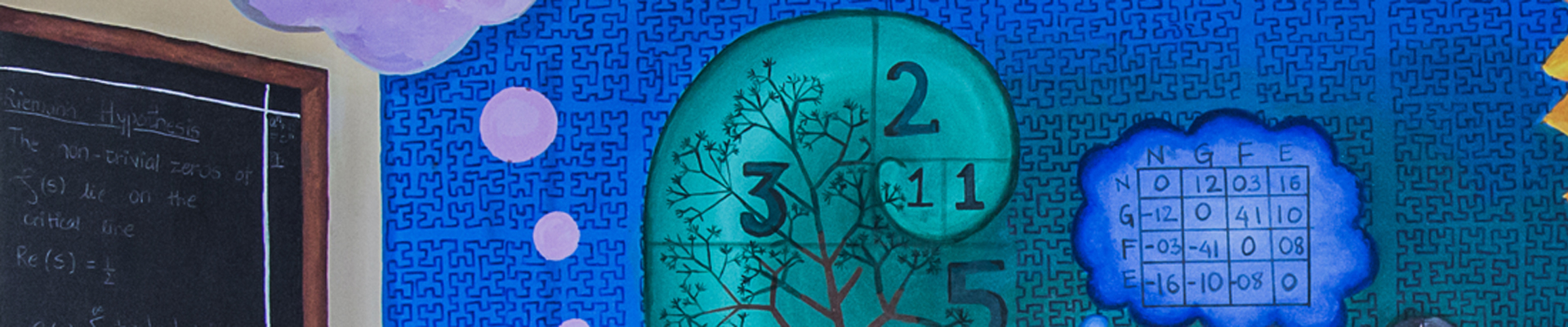# Department of Mathematics

The research activities of the Mathematics department at IISER Pune span a wide range of deep and fundamental areas like algebraic and analytic number theory, automorphic forms, representation theory, finite groups, algebraic K-theory, partial and stochastic differential equations, several variable complex analysis, harmonic analysis, operator theory, algebraic geometry, differential geometry, low dimensional topology, cryptography, algorithms, coding theory, probability theory, mathematical finance and statistical modelling.

## Research Areas

### Algebra, Number Theory and Representation Theory

Topics: Algebraic Number Theory, Automorphic Forms, L-Functions and their Special Values, Arithmetic Geometry, Motives, Galois Representations, Analytic Number Theory, Modular Forms, Theory of Witt vector of associative rings

Finite Group Theory, Linear Algebraic Groups, Lie Groups & Lie Algebras, Representation Theory of Real, p-Adic and Adelic Groups, Cohomology of Arithmetic Groups

Algebraic K-Theory

### Analysis and Differential Equations

Topics: Elliptic Partial Differential Equations, Nonlinear Analysis, Variational Methods, Shape Optimization

Functional Analysis and Operator theory, Nonlinear Dispersive Equations, Nonlocal partial differential equations, Potential theory, Control theory, Equations with measure data

Partial Differential Equations on Riemannian manifolds

Stochastic Differential Equations

Several Complex Variables

Harmonic Analysis on Locally Symmetric Spaces

### Geometry and Topology

Topics: Algebraic Geometry, Vector Bundles, Motivic Cohomology Triangulated Categories, Real Algebraic Geometry, Differential Geometry, Symplectic Geometry

Knot Theory, Low Dimensional Topology

Triangulations, Hyperbolic geometry

Geometric aspects of Locally Symmetric Spaces

### Discrete Mathematics, Cryptography, and Coding Theory

Topics: Cryptography, Combinatorics,  Algorithms

Statistical Designs,  Software Testing

Coding Theory

Spectral Graph Theory

### Applicable Mathematics

Topics: Probability Theory, Mathematical Finance,  Dynamic Game Theory

Queuing Theory,  Stochastic Control,  Large Deviation Theory

Statistical Modeling and Inference

## Centres and Collaborations

The department has been awarded funds to expand research infrastructure under the FIST programme of the Department of Science and Technology. We have an MoU with the Bhaskaracharya Pratishthana, Pune.